# PostgreSQL Joins: Inner, Outer, Left, Right, Natural with Examples

## What are PostgreSQL Joins?

PostgreSQL JOINs are used for retrieving data from more than one tables. With JOINs, it is possible for us to combine the SELECT and JOIN statements into a single statement. A JOIN condition is added to the statement, and all rows that meet the conditions are returned.

The values from different tables are combined based on common columns. The common column mostly is a primary key in the first table and a foreign key of the second table.

In this PostgreSQL tutorial, you will learn:

## Types of Joins

There are two JOIN types in PostgreSQL:

• Inner Joins
• Outer Join

## Inner Joins

There are 3 types of inner joins:

• Theta join
• Natural join
• EQUI join

### Theta Join

A theta join allows one to join two tables based on the condition that is represented by theta. Theta joins can work with all comparison operators. In most cases, the theta join is referred to as inner join.

The theta join is the most basic type of JOIN. It will return all rows from the tables where the JOIN condition is satisfied.

Syntax:

```SELECT columns
FROM table-1
INNER JOIN table-2
ON table-1.column = table-2.column;
```

Consider the following tables of the Demo database:

Book: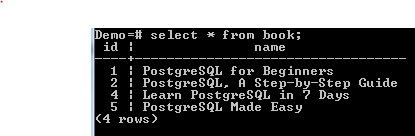Price: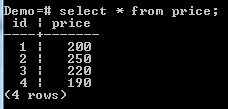We want to see the name of each book and the corresponding Price. We can run the following command:

```SELECT Book.name, Price.price
FROM Book
INNER JOIN Price
ON Book.id = Price.id;
```

This will return the following: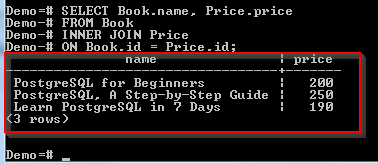Only 3 rows satisfied the join condition.

### EQUI Join

The EQUI join provides us with a way of joining two tables based on primary key/foreign key relationship. For example:

```SELECT *
FROM Book
JOIN Price ON Book.id = Price.id;
```

This will return the following: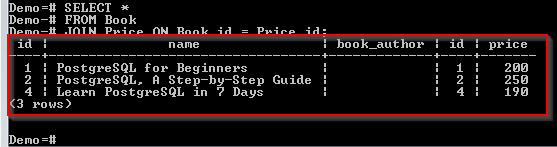Records have been returned from both tables based on the common columns, that is, the id column.

### Natural Join

This type of join provides us with another way of writing an EQUI join. We can improve our previous example by adding the NATURAL keyword as shown below:

```SELECT *
FROM Book
NATURAL JOIN Price;
```

This will return the following: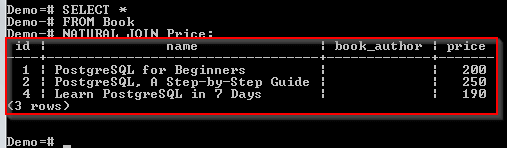Only one id column has been returned. The NATURAL JOIN was able to note that the id column is common in the two tables. Only one was returned.

## Outer Joins

There are three types of outer JOINs in PostgreSQL:

• Left Outer Join.
• Right Outer Join.
• Full Outer Join

### LEFT OUTER JOIN

The LEFT OUTER JOIN will return all rows in the table on the left-hand side and only the rows in the right-hand side table where the join condition has been satisfied.

Syntax:

```SELECT columns
FROM table-1
LEFT OUTER JOIN table-2
ON table-1.column = table-2.column;
```

We need to see the name of each book and the corresponding Price. We can run the following command:

```SELECT Book.name, Price.price
FROM Book
LEFT JOIN Price
ON Book.id = Price.id;
```

This returns the following:All the 4 rows in the Book table have been returned. Only 3 rows from the Price table met the join condition. Hence they were returned. The last book has no corresponding price value.

### RIGHT OUTER JOIN

The RIGHT OUTER JOIN returns all rows in the table on the right-hand side and rows in the table on the left-hand side where the join condition has been satisfied.

Syntax:

.

```SELECT columns
FROM table-1
RIGHT OUTER JOIN table-2
ON table-1.column = table-2.column;
```

For example:

```SELECT Book.name, Price.price
FROM Book
RIGHT JOIN Price
ON Book.id = Price.id;
```

This returns the following: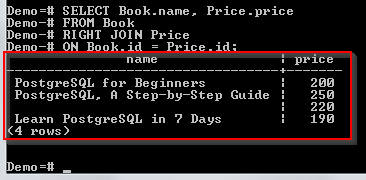All the rows in the Price table have been returned. Only the rows in the Book table that met the join condition were returned. The 3rd row has no value for name since no match was found.

### Full Outer Join

This type of JOIN will return all rows in the table on the left-hand side and all rows in the table on the right-hand side with nulls where the join condition is not satisfied.

Syntax:

```SELECT columns
FROM table-1
FULL OUTER JOIN table-2
ON table-1.column = table-2.column;
```

For example:

```SELECT Book.name, Price.price
FROM Book
FULL OUTER JOIN Price
ON Book.id = Price.id;
```

This returns the following:All rows from all tables have been returned, with nulls where no match was found.

## Inner Joins

### Theta Join

Step 2)

1. From the navigation bar on the left- Click Databases.
2. Click Demo.Step 3) Type the query in the query editor:

```SELECT Book.name, Price.price
FROM Book
INNER JOIN Price
ON Book.id = Price.id;
```

Step 4) Click the Execute button.It should return the following: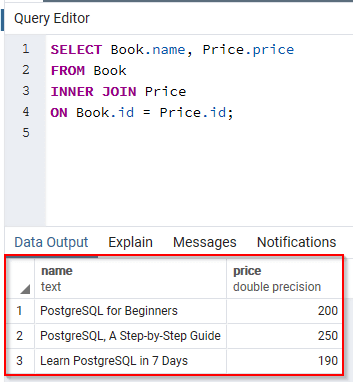### EQUI Join

Step 2)

1. From the navigation bar on the left- Click Databases.
2. Click Demo.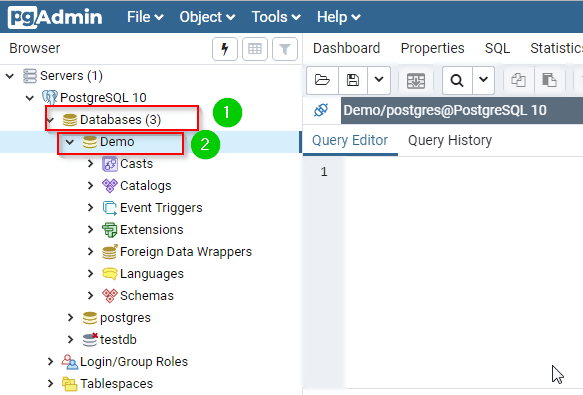Step 3) Type the query in the query editor:

```SELECT *
FROM Book
JOIN Price ON Book.id = Price.id;
```

Step 4) Click the Execute button.It should return the following: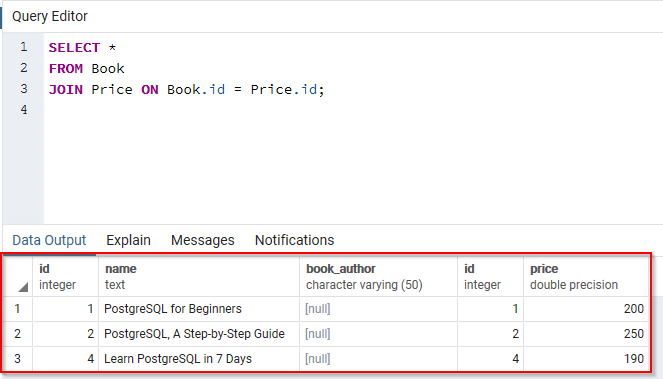### Natural Join

Step 2)

1. From the navigation bar on the left- Click Databases.
2. Click Demo.Step 3) Type the query in the query editor:

```SELECT *
FROM Book
NATURAL JOIN Price;
```

Step 4) Click the Execute button.It should return the following:### INNER JOIN (simple join)

Step 2)

1. From the navigation bar on the left- Click Databases.
2. Click Demo.Step 3) Type the query in the query editor:

```SELECT Book.name, Price.price
FROM Book
INNER JOIN Price
ON Book.id = Price.id;
```

Step 4) Click the Execute button.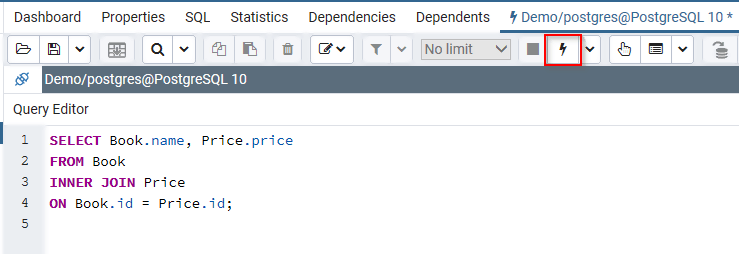It should return the following:## Outer Joins

### LEFT OUTER JOIN

Step 2)

1. From the navigation bar on the left- Click Databases.
2. Click Demo.Step 3) Type the query in the query editor:

```SELECT Book.name, Price.price
FROM Book
LEFT JOIN Price
ON Book.id = Price.id;
```

Step 4) Click the Execute button.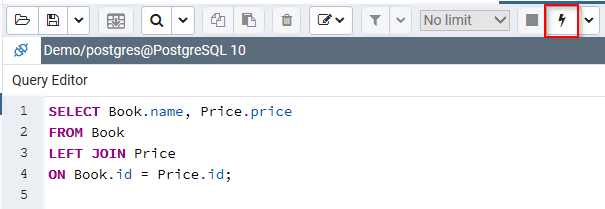It should return the following:### RIGHT OUTER JOIN

Step 2)

1. From the navigation bar on the left- Click Databases.
2. Click Demo.Step 3) Type the query in the query editor:

```SELECT Book.name, Price.price
FROM Book
RIGHT JOIN Price
ON Book.id = Price.id;
```

Step 4) Click the Execute button.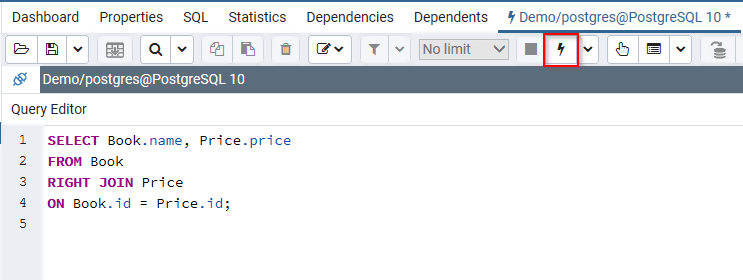It should return the following: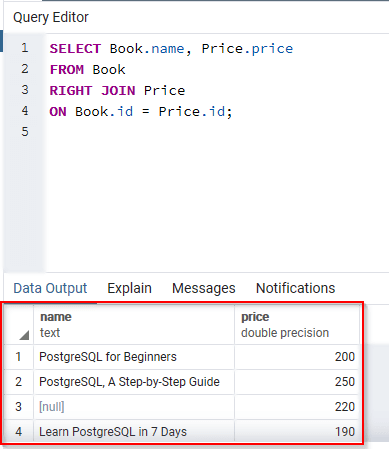### Full Outer Join

Step 2)

1. From the navigation bar on the left- Click Databases.
2. Click Demo.Step 3) Type the query in the query editor:

```SELECT Book.name, Price.price
FROM Book
FULL OUTER JOIN Price
ON Book.id = Price.id;
```

Step 4) Click the Execute button.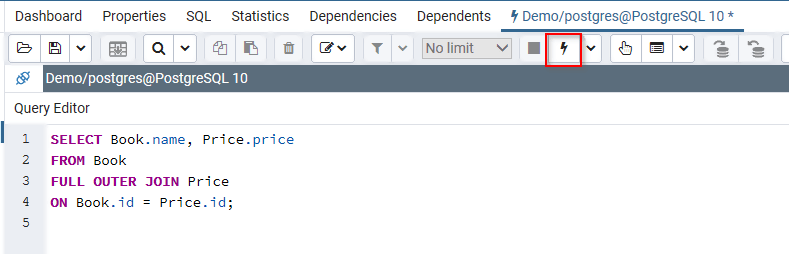It should return the following: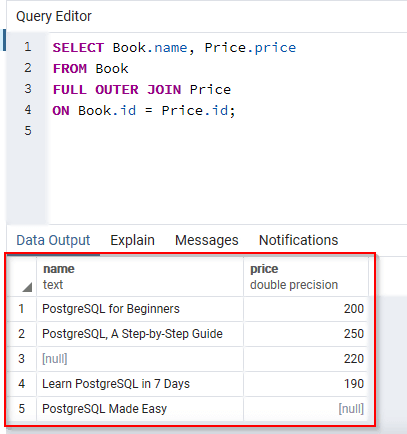### Summary:

• In PostgreSQL, we use JOINs when we need to retrieve values from more than one table.
• The INNER JOIN is the most basic type of JOIN. It returns all records where the specified JOIN condition was satisfied.
• The LEFT OUTER JOIN returns all rows in the left-hand table and only the rows in the other table where the join condition has been satisfied.
• The RIGHT OUTER JOIN returns all rows in the right-hand table and only rows in the other table where the join condition has been satisfied.
• This type of JOIN returns all rows in the left-hand table and all rows in the right-hand table with nulls where the join condition is not satisfied.